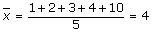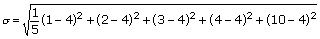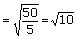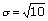## Definition Of Variance

Variance is a statistical measure that tells us how measured data vary from the average value of the set of data.

In other words, variance is the mean of the squares of the deviations from the arithmetic mean of a data set.

Variance is the square of the standard deviation.
The formula for variance is### Solved Example on Variance

#### Ques: Find the variance of the data set {1, 2, 3, 4, 10}.

##### Choices:

A. 10
B. 9
C. 8
D. 7
tep 1: The mean of the data set {1, 2, 3, 4, 10} is[Use the formula for mean.][Use the formula for mean.]
Step 3:Step 4: The variance of the data set is[Substitute]